# Catch up on the latest AI articles# Does The Quality Of Recommendations Received By Different People Change? Addressing Bias In Recommendation Systems

3 main points
✔️ Proposed 4 approaches to distinguish between mainstream and niche users
✔️ Demonstrate a bias that mainstream users are more accurate than niche users in traditional recommendation models
✔️ Suggest 3 methods to eliminate bias

written by Ziwei ZhuJames Caverlee
(Submitted on 15 February 2022)

Subjects: Information Retrieval (cs.IR)code：The images used in this article are from the paper, the introductory slides, or were created based on them.

## first of all

The recommendation system plays an important role in terms of connecting users with better items to alleviate the problem of information overload.

Most recommendation systems, including the old linear models and more recent neural net models, make recommendations by predicting user preferences based on collaborative filtering (CF).
The key idea of CF is to infer preferences from the behavior of a user by finding users with similar interests as the target user. Therefore, the quality of recommendation depends heavily on how easily the recommendation model can find similar users.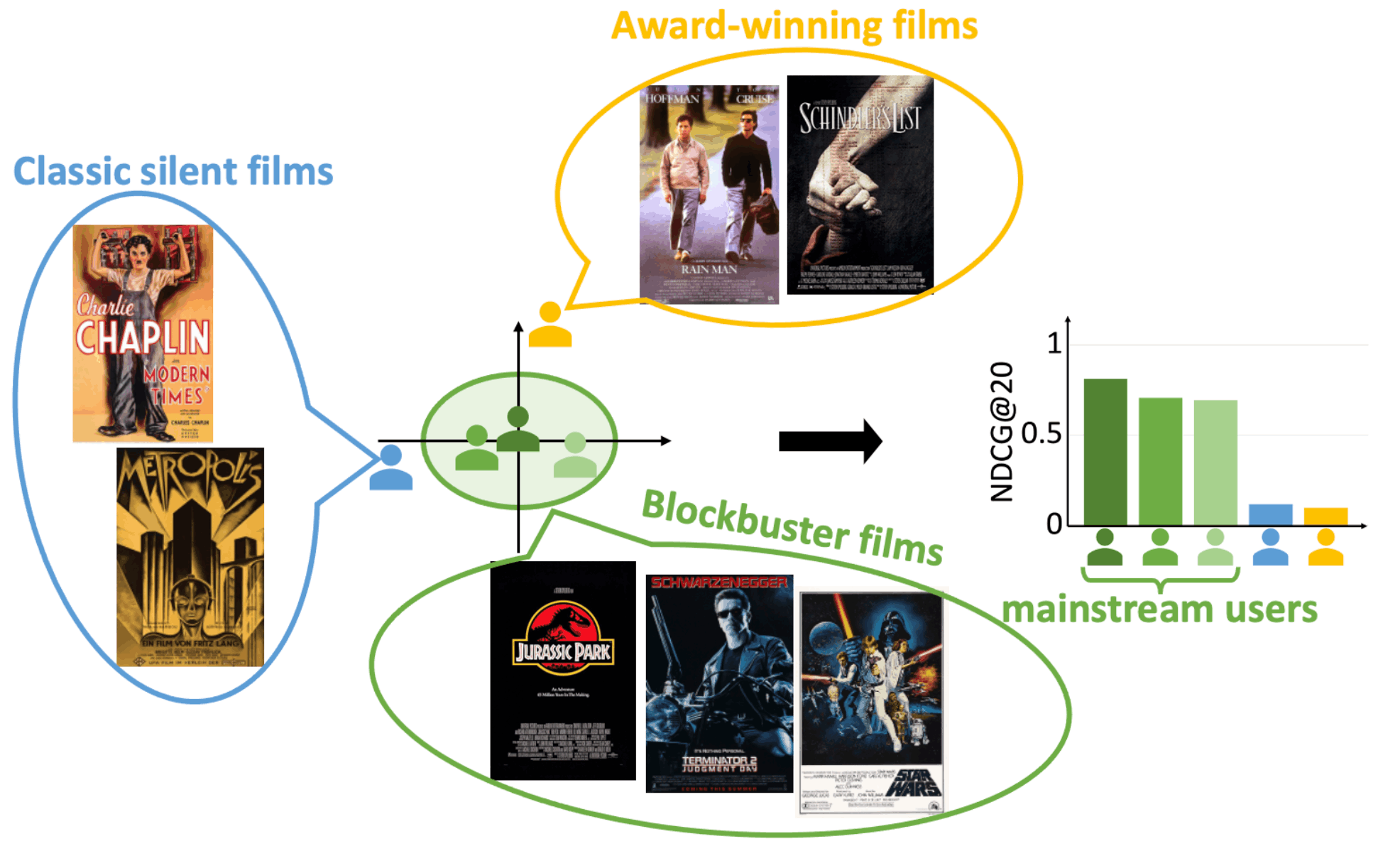The above figure shows three mainstream users and two niche users. three mainstream users tend to prefer popular blockbusters and have high recommendation accuracy (NDCG@20) in the recent variational autoencoder (VAE) model. On the other hand, two niche users (one who prefers older silent films and one who prefers award-winning films from the late 80s) have lower recommendation accuracy.

Non-mainstream niche users have a harder time finding similar users, which tends to result in lower quality recommendations.
This tendency for recommendation models to favor mainstream users over niche users is called mainstream bias.

## Calculating a user's Mainstream Score

The authors first proposed the mainstream score, which indicates how mainstream each user is, to analyze the impact of mainstream bias on recommendations. To calculate the mainstream score, four methods based on outlier detection techniques are considered.

Let's look at each of the four methods one by one.

### Similarity-based

In the similarity-based approach, the more similar users are to the target user, the higher the main stream score.
For all user pairs, the similarity between users is calculated by Jaccard similarity. Here, the similarity between users is denoted by $J_{u,v}$.
The average similarity between the target user $u$ and another user $v$ is the mainstream score.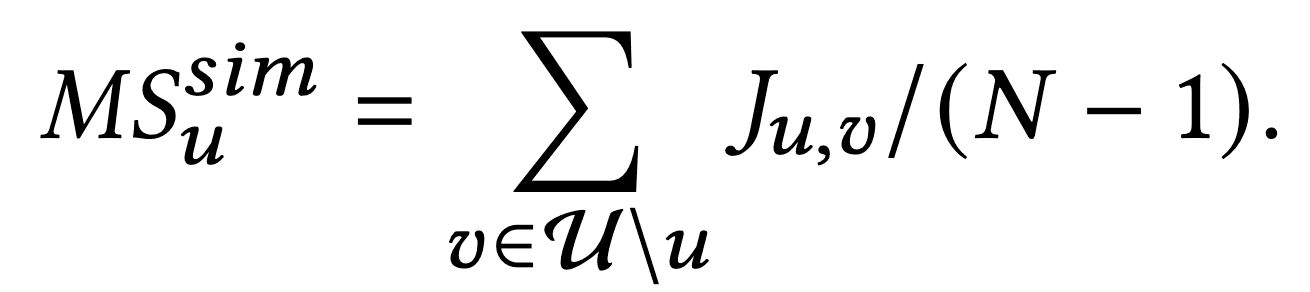### Density-based

The density-based approach determines if a sample is an outlier based on the density of its neighborhood. In this study, the well-known Local Outlier Factor Method (LOF) is used to identify outliers (niche users); LOF has a negative value because a higher value indicates that the sample is an outlier.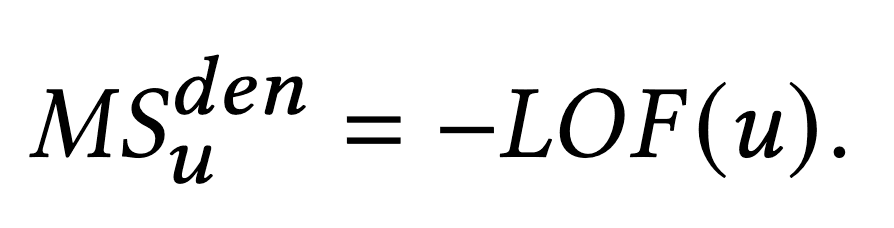### Distribution-based

In the distribution-based approach, we calculate the probability of an item being preferred based on the similarity between the distribution vector $d$ and the history vector $O_u$ of items preferred by the target user. In this case, $d$ is the average of all users' item preference histories. Also, $cos()$ represents the cosine similarity.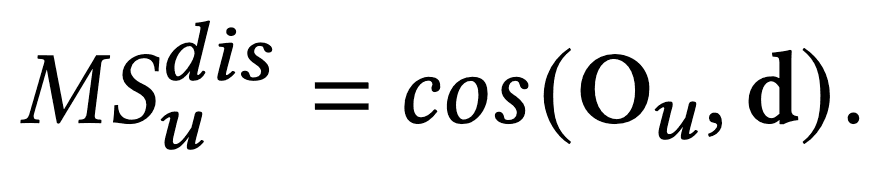### DeepSVDD-based

DeepSVDD is a deep learning based outlier algorithm Deep Support Vector Data Description. It maps sample data to a hypersphere by a neural network and considers samples far from the center of the hypersphere as outliers. Here $DeepSVDD\left(O_{u}\right)$ is a vector of user $u$ on the hypersphere and $c$ is a vector representing the center of the hypersphere.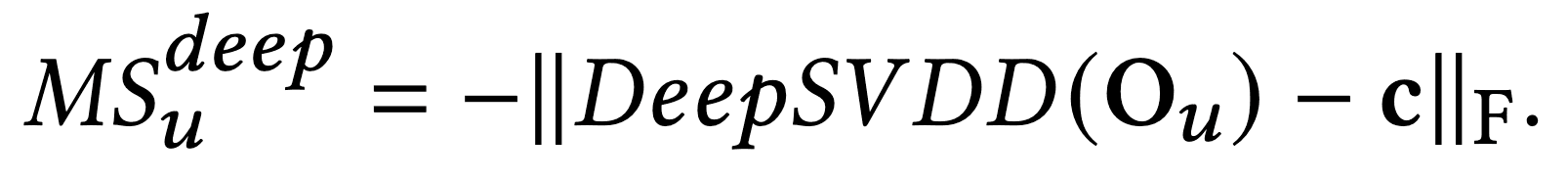## Demonstrating Mainstream Bias

To observe the mainstream bias, we sort the users in ascending order based on the mainstream score from earlier and divide them into five subgroups of equal size.

The table below shows the results of applying VAE to MovieLens 1M and averaging the NDCG@20 for each subgroup. Here, 'low', 'med-low', 'medium', 'med-high', and 'high' are the groups with the highest mainstream scores.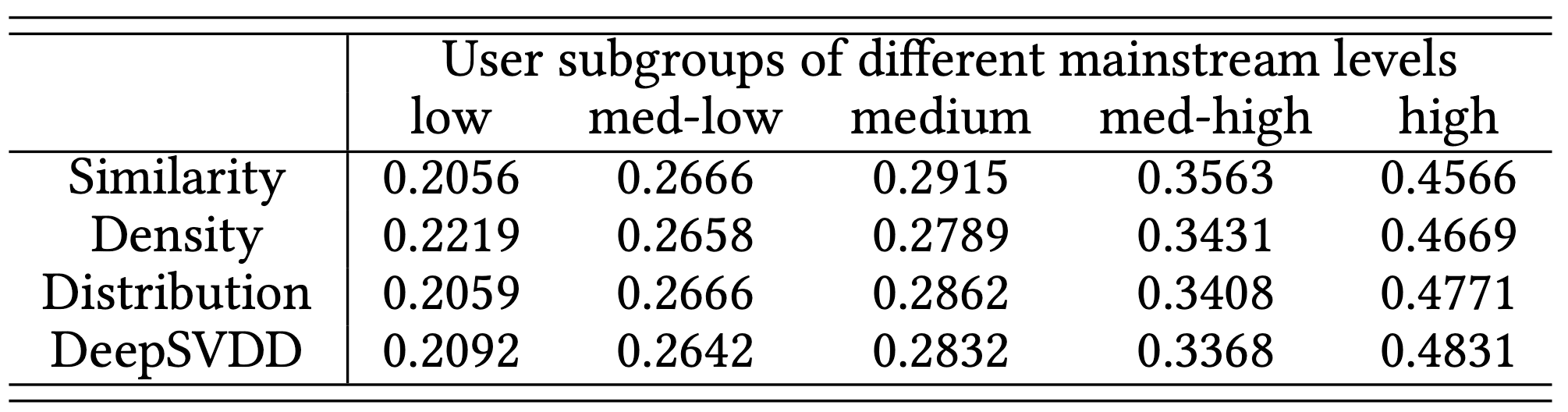From the table, we can see that for all four approaches, the group with the higher mainstream score has better recommendation accuracy.

The results show that all the approaches proposed by the authors can identify niche users that result in low recommendation accuracy, and that the recommendation models create a serious mainstream bias.

The authors have also experimented with other models such as MF, BPR, and LOCA, as well as other datasets such as Yelp and Epinions, and confirmed that the same trend is observed.

## Proposed Solution

To mitigate the mainstream bias, we propose two global methods and one local method.
The global method is to train a single model by increasing the weight of niche users when training models, while the local method is to train local models customized for different users.

### global method

Distribution Calibration Method (DC)

DC is a data augmentation-based approach that generates synthetic data similar to existing niche users so that the niche users become mainstream in the training dataset.

Specifically, we first identify niche users using one of the approaches described above. For example, in the case of DeepSVDD, we consider the 50% of users with the lowest mainstream score to be niche users. We then obtain a calibrated distribution vector $p_u$ by obtaining similar users for a given niche user $u$.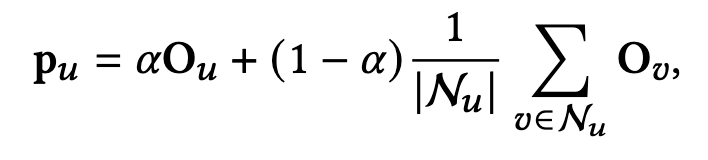Inthis case,$\mathcal{N}_u$ is the set of similar users calculated by Jaccard similarity and $0 \leq \alpha \leq 1$ is a hyperparameter that controls the weight of user $u$'s preference history.

Finally, we sample users based on this distribution vector $p_u$. Models trained with this extended dataset allow us to increase the importance of niche users and mitigate the mainstream.

Weighted Loss Method (WL)

WL is a way to directly increase the weights of niche users in the loss function; taking the VAE model as an example, the loss function is as follows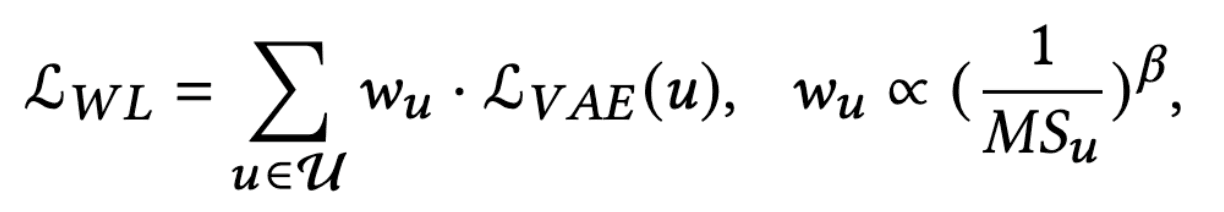where $\mathcal{L}_{V A E}(u)$ is the original VAE loss for user $u$ and $w_u$ is the weight of user $u$. The weight for $u$ is $\left(\frac{1}{M S_{u}}\right)^{\beta}$, where $\beta$ is a hyperparameter that controls the strength of bias removal. The larger $\beta$ is, the stronger the bias removal works, and 0 means no removal.

This weighted loss function allows us to increase the importance of niche users.

### local method

The global approach may have a trade-off where the recommendation accuracy of niche users is higher, but the accuracy of mainstream users is lower. Therefore, the author proposes a local approach.

Local Fine Tuning(LFT)

We propose to use local recommendation methods to deal with the main stream bias. The idea of the local recommendation method is to select anchor users and train a specialized anchor model for each anchor user.

LFT is divided into three steps.

1. Train the base model $\phi$ using all datasets
2. Neighboring users whose preferences are similar to the target user $u$. From $\mathcal{N}_{u}$, a sub-dataset of preference history data only Create $O_{\mathcal{N}_{u}}=\left\{O_{v} \mid v \in \mathcal{N}_{u}\right\}$.
3. Fine-tune the base model for user $u$ using the sub-dataset $O_{\mathcal{N}_{u}}=\left\{O_{v} \mid v \in \mathcal{N}_{u}\right\}$.

Thus, by using a local model $\phi_{u}$ for fine-tuned $u$, niche users can be less influenced by mainstream users and other niche users.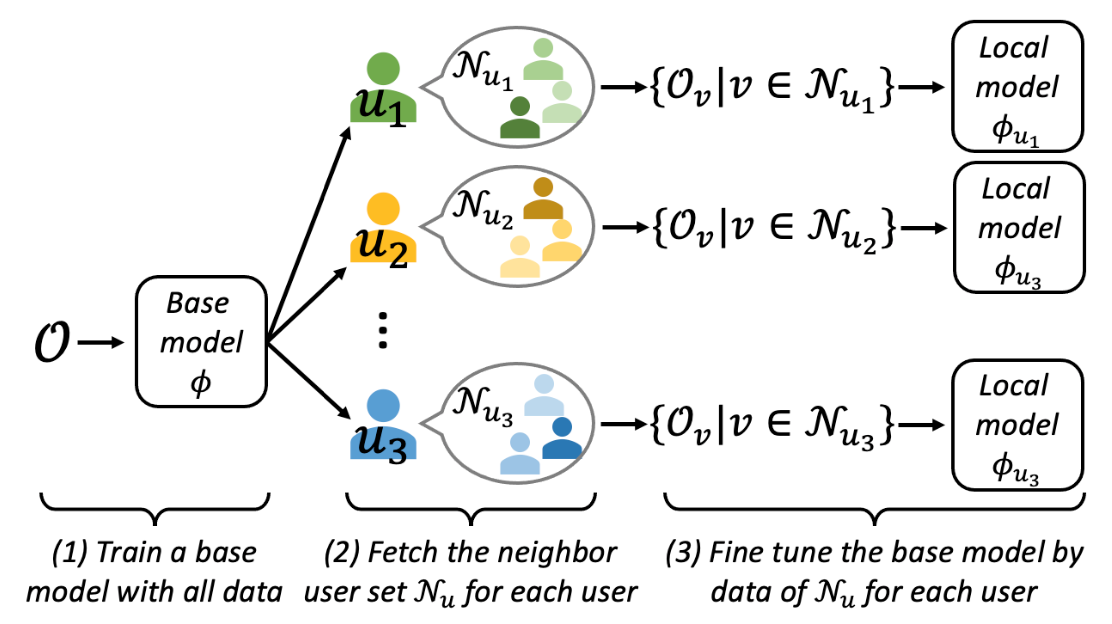## experiment

The experiments are conducted on ML1M, Yelp and Epinions datasets.
VAE is adopted as the base model and the experiments are conducted with the model in which the proposed method is applied to VAE. The global methods are Distribution Calibration (DC) and Weighted Loss (WL). For local methods, we use the latest Local Collaborative Autoencoders (LOCA) as a baseline, the proposed method Local Fine Tuning (LFT) and its ensemble version, the Ensemble version of the LFT model ( EnLFT).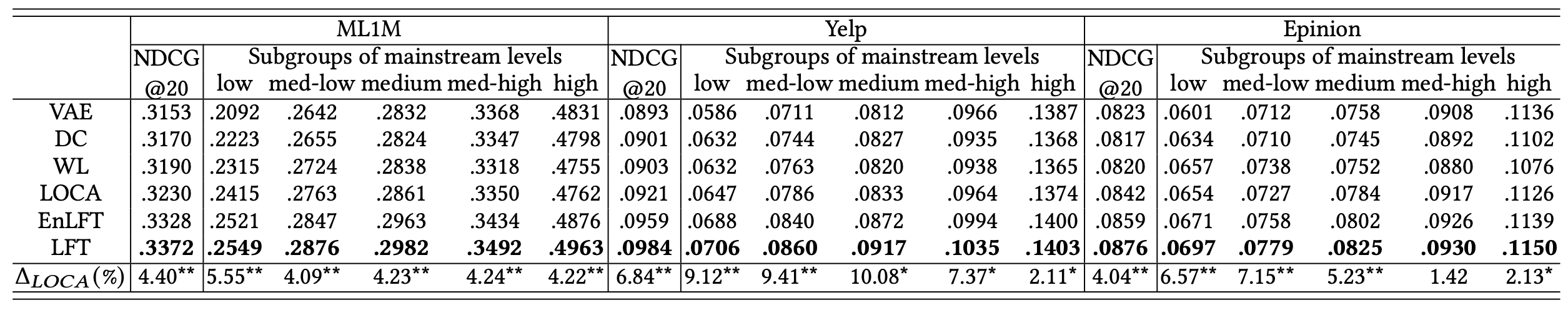The results show that the proposed LFT achieves excellent accuracy NDCG@20, which is significantly better than VAE for the 'low', 'med-low' and 'medium' user groups. The accuracy is also improved for the more mainstream user groups such as 'med-high' and 'high'.
This shows that LFT is effective not only for niche users but also for mainstream users.

DC and WL, which are global methods, are more accurate for niche users, but less accurate for mainstream users.

## summary

How was it? We introduced a method for calculating the mainstream score, a demonstration of mainstream bias, and three suggested methods for mitigating the bias. We felt that focusing on the main stream bias, in which mainstream users benefit from the recommendation system while niche users do not benefit sufficiently, is an important perspective for realizing a fair recommendation system.

We hope that bias will be studied further for impartial recommender systems in the future.

If you have any suggestions for improvement of the content of the article,